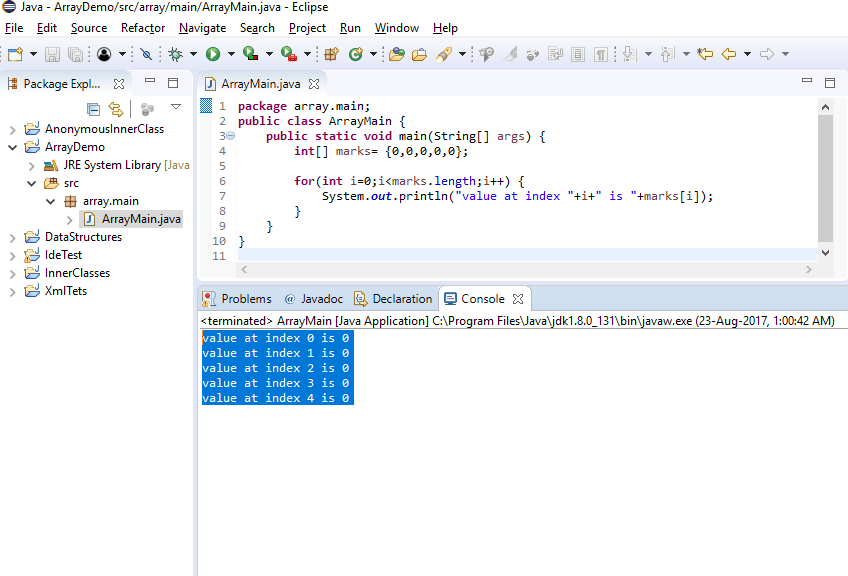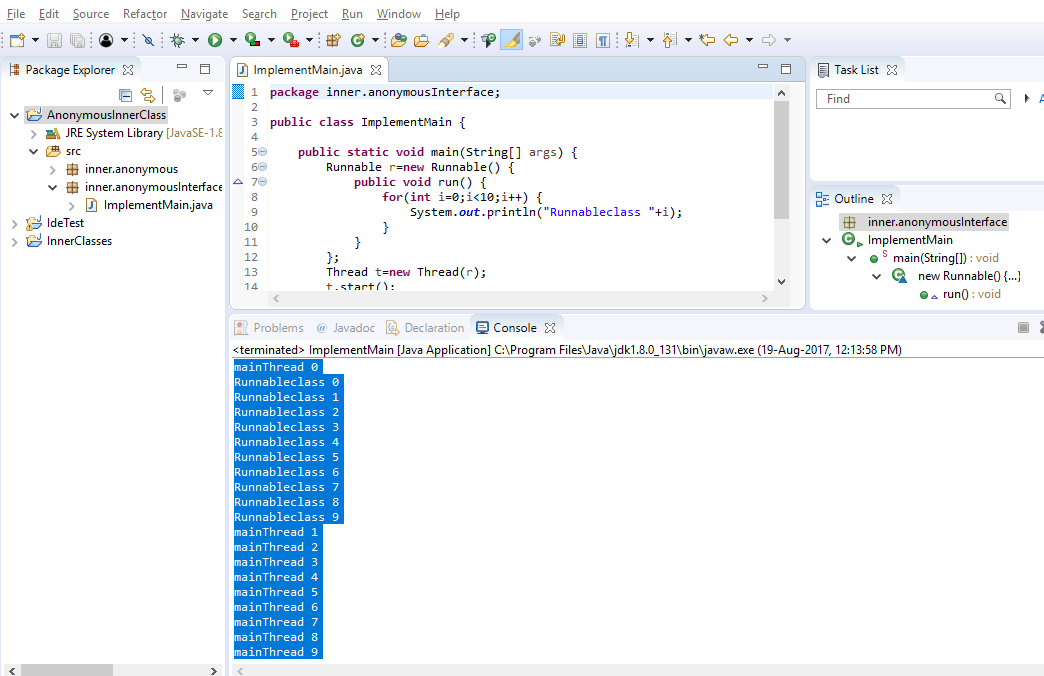Home ProgrammingJava Java primitive Array

# Java primitive Array

705 views

## Introduction

We have discussed about the primitive array in java in previous posts also.

An primitive array is group of variables of same type referenced by common name. Each value inside array are Elements of that array. Number of elements of array is size of that array. Array is most convenient way to store group of elements of same type.

## Types Of Array

Simplest type of primitive array is One-Dimensional(1D) array which contain series of values of same type. Array of multiple 1D array is Two-Dimensional array, array of multiple 2D array is Three-Dimensionl array and so on. Now we can conclude that an Element of any n-Dimensional array will be a N-1 Dimensional array.

## One Dimensional Array

Here I will first describe about One-Dimensional array.

### General form of Declaration

`type arrayname[];`
Here type is base type that may be primitive or non-primitive data type of java representing the type of each and every element in java.
Eg. `int marks[];`
Here marks is an array containing elements of type int.

### Creating an array:

Creating a java primitive array means allocating memory for all elements of array. We can use new operator to create array.
General syntax for creating an array is :
`arrayname = new type[size];`
Here size is integer representing number of elements in array. Type must be same as that in declaration statement.
Eg. `marks= new int;`
Here marks is array of 5 integers.

### Accessing array elements:

After this statement, we can access any elements of array using arrayname followed by index inside square brackets. By default, Index will be started from 0. All the elements of array are initialized to default value. In the case of marks all the elements will be 0;
Eg. `marks = 26;`
`System.out.println(“first element ” + marks + ”| Second elements ”+marks);`

### Array Initializer:

An array initializer is a list of comma separated expressions surrounded by curly braces.
Eg. `{23,43,26,47,36}`
We can initialize array by using array initializer expression only at the time of declaration. In this case new operator is not required and size of array will be equals to number of items inside initializer expression. Java not allowed to initialize array using Array Initializer once you declared the array.

### Some valid array creations:

1. `int marks[]; marks=new int;`
2. `int marks[]=new int;`
3. `int []marks=new int;`
4. `int []marks= {0,0,0,0,0};`
5. `int marks[]= {0,0,0,0,0};`
6. `int[] marks= {0,0,0,0,0};`

Above all lines of code do same thing. These all will create an array named `marks`, which can store 5 `int` values. And these all values would be 0.

### Example:

```package array.main;
public class ArrayMain {
public static void main(String[] args) {
int[] marks= {0,0,0,0,0};

for(int i=0;i<marks.length;i++) {
System.out.println("value at index " + i + " is " + marks[i]);
}
}
}
```

### OutputOutput primitive array

value at index 0 is 0
value at index 1 is 0
value at index 2 is 0
value at index 3 is 0
value at index 4 is 0

We will discuss about multi-dimensional arrays in next article. Happy Learning!

0 comment

This site uses Akismet to reduce spam. Learn how your comment data is processed.

## Java primitive Array

by Log Raj time to read: 2 min